# ISEE Upper Level Math : How to find out if a number is prime

## Example Questions

← Previous 1

### Example Question #1 : How To Find Out If A Number Is Prime

Add all of the prime numbers between 50 and 70.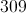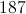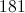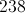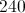Explanation:

The prime numbers between 50 and 70 are 53, 59, 61, and 67. Their sum is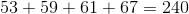.

### Example Question #2 : How To Find Out If A Number Is Prime

Add all of the prime numbers between 20 and 40.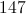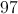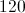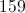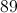Explanation:

The prime numbers between 20 and 40 are 23, 29, 31, and 37.

Their sum is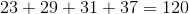.

### Example Question #3 : How To Find Out If A Number Is Prime

Which of these numbers is prime?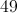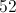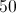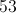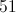Explanation:

A prime number has exactly two factors, 1 and itself. We can eliminate four choices by finding other factors: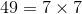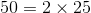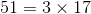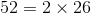53 has only 1 and 53 as factors, so it is the only prime among the choices.

### Example Question #4 : How To Find Out If A Number Is Prime

How many composite numbers are between 61 and 80 inclusive?Explanation:

There are twenty integers from 61 to 80 inclusive. Counting composite numbers can be made easier by weeding out the primes - 61, 67, 71, 73, 79. Removal of these five primes leave fifteen composite numbers.

### Example Question #5 : How To Find Out If A Number Is Prime

Which of the following numbers is prime?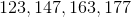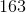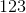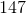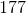Explanation:

The correct answer is, and this can be determined in the following manner.

First, find the approximate square root of the number: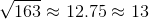We know this because: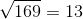Therefore, we only need to consider prime numbers through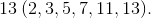Isevenly divisible by any of these numbers? In this case, the answer is no, thereforeis prime. Consider the case where the answer is not prime:.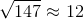We know this because: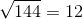Therefore, we need to consider the followig prime numbers: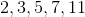Isdivisible by any of these numbers? In this case, the answer is yes.is divisible by.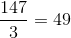### Example Question #6 : How To Find Out If A Number Is Prime

How many composite numbers are betweenandinclusive?Explanation:

There are twenty integers fromtoinclusive. Counting composite numbers can be made easier by weeding out the primes -. Removal of these five primes leavescomposite numbers.

### Example Question #7 : How To Find Out If A Number Is Prime

Which of the following numbers is prime?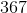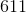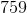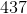Explanation:

The correct answer to this question is. To determine if a number is prime, first find the approximate square root of the number: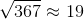Next, determine if the number is divisible by any prime numbers up to and including the square root. In this instance consider the numbers: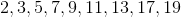is not divisible by any of the above numbers; therefore, it is prime.

### Example Question #8 : How To Find Out If A Number Is Prime

How many composite numbers are between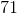andinclusive?Explanation:

There are thirty integers fromto.  Counting composite numbers can be made easier by weeding out the primes -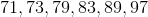. There are six primes in this range, so this leaves twenty-four composite numbers.

### Example Question #9 : How To Find Out If A Number Is Prime

Reduce the fraction: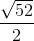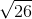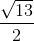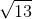Explanation:First, given that 13 times 4 is 52, the numerator can be simplified by taking the square root of 4 and multiplying it by the square root of 13.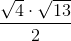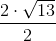### Example Question #10 : How To Find Out If A Number Is Prime

How many prime numbers are there between 1 and 20?

11

2

8

9

7